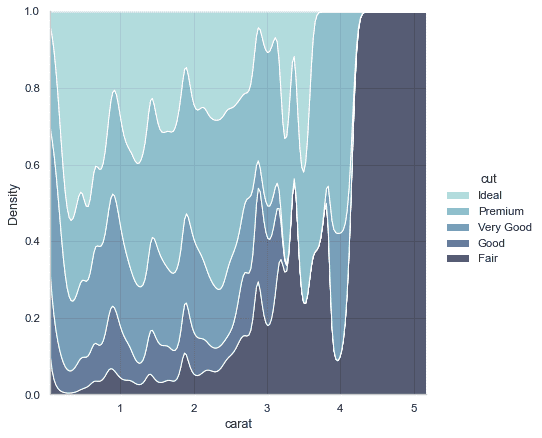数学建模与计算
Problem 1.

This gives the height of the shuttlecock above the ground as
$$A=\alpha_{2}-\frac{g}{\alpha_{1}} t+\frac{g}{\alpha_{1}^{2}}\left(1-\exp \left(-\alpha_{1} t\right)\right)$$
where $\alpha_{2}$ is the initial height.
(a) Check these solutions.
(b) Suppose that heights are measured at various times and we wish to use the data to calculate $\alpha_{1}$ and $\alpha_{2}$. Assume the data satisfies $y=A(x, t)+e$, where $A$ is the function given above and $x=\left[\begin{array}{l}\alpha_{1} \ \alpha_{2}\end{array}\right]$ and $e$ is normal with constant standard deviation. Write Matlab files for $A(x, t)$ and the Jacobian. Check that they work!
(c) Data for $\mathrm{t}$ and $\mathrm{Y}$ values can be found using load(tut6.mat). Use the data to estimate $\alpha_{1}$ and $\alpha_{2}$.
(d) Estimate the covariance matrix of $\alpha_{1}$ and $\alpha_{2}$.
(e) Plot the data, the least squares fit, and the fit $\pm$ three standard deviations. Comment on the error estimates shown in your graph.

Proof .

Problem 2.

The velocity $v$ of a falling shuttlecock is modelled by
$$\frac{d v}{d t}=g-\alpha_{1} v, \quad v(0)=0$$
where $g=9.8 \mathrm{~ms}^{-2}$ and $\alpha_{1}$ is the drag coefficient of the shuttlecock.
The solution of this is
$$v=\frac{g}{\alpha_{1}}\left(1-\exp \left(-\alpha_{1} t\right)\right.$$

Proof .

Problem 3.

Consider the function
$$\Psi(\mathbf{x})=x_{1}^{4}+x_{1} x_{2}+x_{1}+x_{2}^{2}$$
(a) Calculate $\nabla \Psi$ and the Hessian.
(b) Starting with $\mathbf{x}_{0}=\left[\begin{array}{l}1 \ 1\end{array}\right]$, find the location of the minimum of $\Psi$ t. using the steepest descent method. How many iterations are requ
(c) Repeat the previous question using the Newton-Raphson search r

Proof .

Problem 4.

Download ass2q1.mat from Canvas and load it into Matlab. The file contains 20 data points for $t$ and $y$ for the model
$$y=a_{0}+a_{1} t+e .$$
Let $x=\left[\begin{array}{l}a_{0} \ a_{1}\end{array}\right]$ be the vector of parameters.
(a) Assuming that $\Gamma_{e}=\sigma_{e}^{2} \mathbf{I}$, where $\sigma_{e}$ is constant, calculate $\hat{x}{l s}$ and $\Gamma{\hat{r}{b} \text { . }}$ (b) Plot the observed data $(t, y)$ with prediction $\hat{y}$ from part a) and the confidence interval $\hat{y} \pm 3 \sigma{\hat{j}}$. Make sure the observed data, prediction and the interval are clearly distinguishable in your graph.
(c) As you may have noticed from the graph, the variance of $e$ is not same for all of the observations; if we let $\operatorname{var}\left(c_{1}\right)=\sigma_{c}^{2}$, then $\operatorname{var}\left(e_{10}\right)=\operatorname{var}\left(\mathrm{e}{20}\right)=5 \sigma{c}^{2}$ and $\operatorname{var}\left(c_{i}\right)=\sigma_{c}^{2}$ for
the rest of the observations, write the general form of $\Gamma_{e}$ in this case.
(d) Calculate the Gauss-Markov estimates $\hat{x}{g m}$ and $\Gamma{z_{g m}}$.
(e) Plot the observed data and the new predictions with new $\pm 3 \sigma$ confidence intervals.

Proof .数学建模代写认准UpriviateTA

BS equation代写